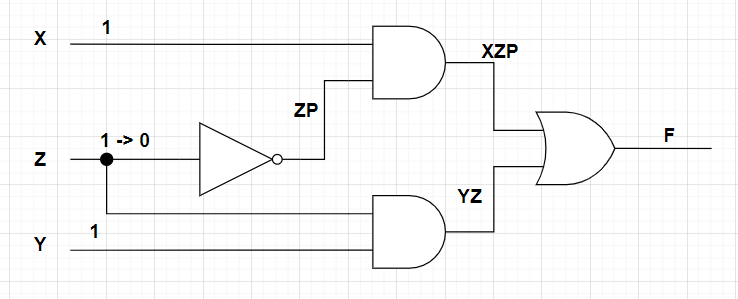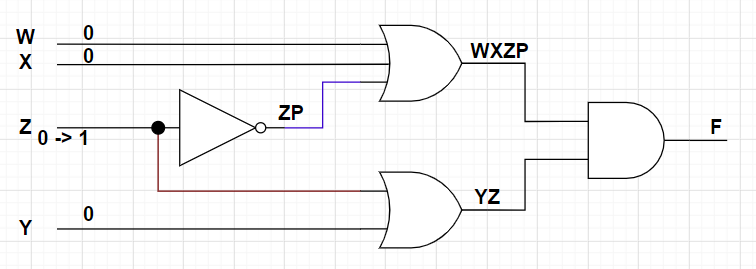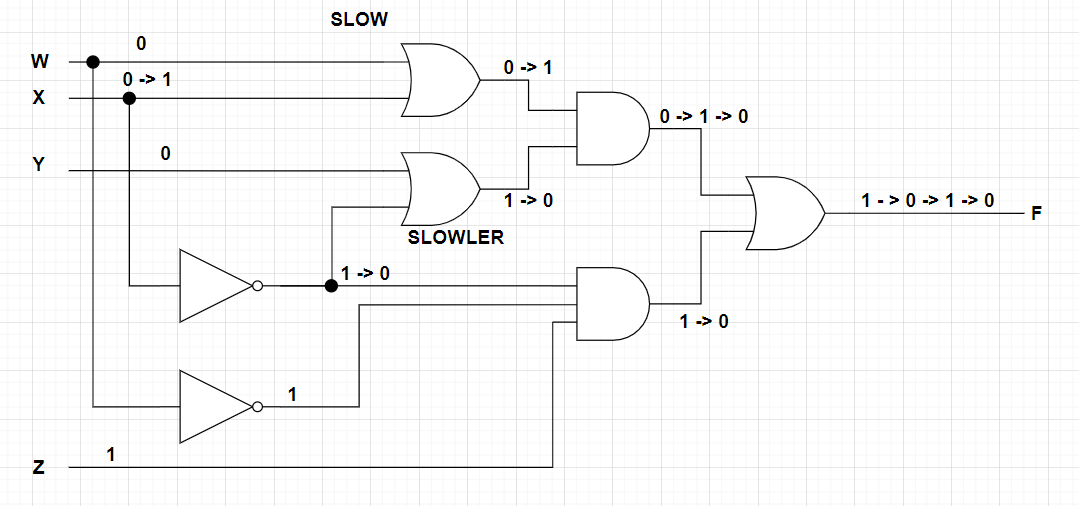# FPGA及硬件设计中的竞争与冒险

### 1 竞争

• 布线延迟造成竞争Verilog 逻辑为： assign OUT = A & B;  （假设 A,B 赋值为：A = 1, B= 0；A= 0, B = 1；）

 input A input B output OUT 0 0 0 0 1 0 1 0 0 1 1 1

• #### 逻辑延迟### 2 冒险

out = a & b & c;  (c =0, a =x, b =x); （1）

out = a & b & c;  (c =1, a =x, b =x);  （2）

• #### 冒险分类：

静态冒险有分为：

• 静态1型冒险X = 1; Y = 1; Z = 1 -> 0 ； 输入有一个变量发生变化， ZP 是Z的反向， 所以Z 和 ZP之间存在时间差，最终在XZP 和 YZ 之间存在时间差，经过或门后 这个时间差将产生毛刺，因此。

• 静态0型冒险always @ (posedge clk or posedge F)

if(F)…..

else …..

• 动态冒险

wire WX = W | X;

wire XY = Y | (~X);

wire WXY = WX & XY;

wire WXZ = (~W) & (~X) & Z;

assign F = WXY | WXZ;• 异步信号输入

### 3 冒险消除方法

1）增加滤波电容：组合电路逻辑输出到片外。可以使用滤波电容来消除毛刺，从而避免冒险。2）使用选通信号，消除毛刺输出3）修改逻辑设计• 增加冗余设计: 利用冗余项消除毛刺函数式和真值表所描述的是静态逻辑，而竞争则是从一种稳态到另一种稳态的过程。因此竞争是动态过程，它发生在输入变量变化时。此时，修改卡诺图，增加多余项，在卡诺图的两圆相切处增加一个圆，可以消除逻辑冒险。
• 采用选通电路: 通过控制选通电路的时间（位置），可以有效的避免由于布线等原因产生的毛刺，即有毛刺的地方不选通。 比如时序电路中的posedge clk 也可以有效去除毛刺， 只要cnt + 1 在一个时钟周期内可以稳定输出

reg [7:0] cnt = 0;

always @ (posedge clk)

cnt <= cnt + 1;

• 采用可靠性编码，如格雷码 。 比如状态机 st = 00, 01, 10, 11    格雷码状态机： 00, 01 , 11, 10.

### 4 检查电路是否存在竞争冒险1）相切的卡诺图有竞争冒险

2）使用示波器测量

3）使用后仿真进行仿真

5 常见的出现竞争冒险

```reg [1:0] cs = 0;
reg [1:0] ns = 0;

always @ (posedge clk)
if(reset) cs <= 0;
else cs <= ns;

reg q = 0;
always @ (*)
case (ns)
0:
begin
q = 1;
ns = cs + 1;
end
1:
begin
q = 0;
ns = cs + 1;
end
2:
begin
q = 0;
ns = cs + 1;
end
3:
begin
q = 1;
ns = cs + 1;
end
endcase```

```reg [1:0] st = 0;

always @ (posedge clk)
if(reset) st <= 0;
else
case (st)
0:
begin
q <= 1;
st <= st + 1;
end
1:
begin
q <= 0;
st <= st + 1;
end
2:
begin
q <= 0;
st <= st + 1;
end
3:
begin
q <= 1;
st <= st + 1;
end
endcase
```

always @ (*)

cnt = cnt + 1;

always @ (posedge clk)

cnt <= cnt + 1;

Posted in FPGA, FPGA 教材教案, Verilog, 教材与教案, 文章